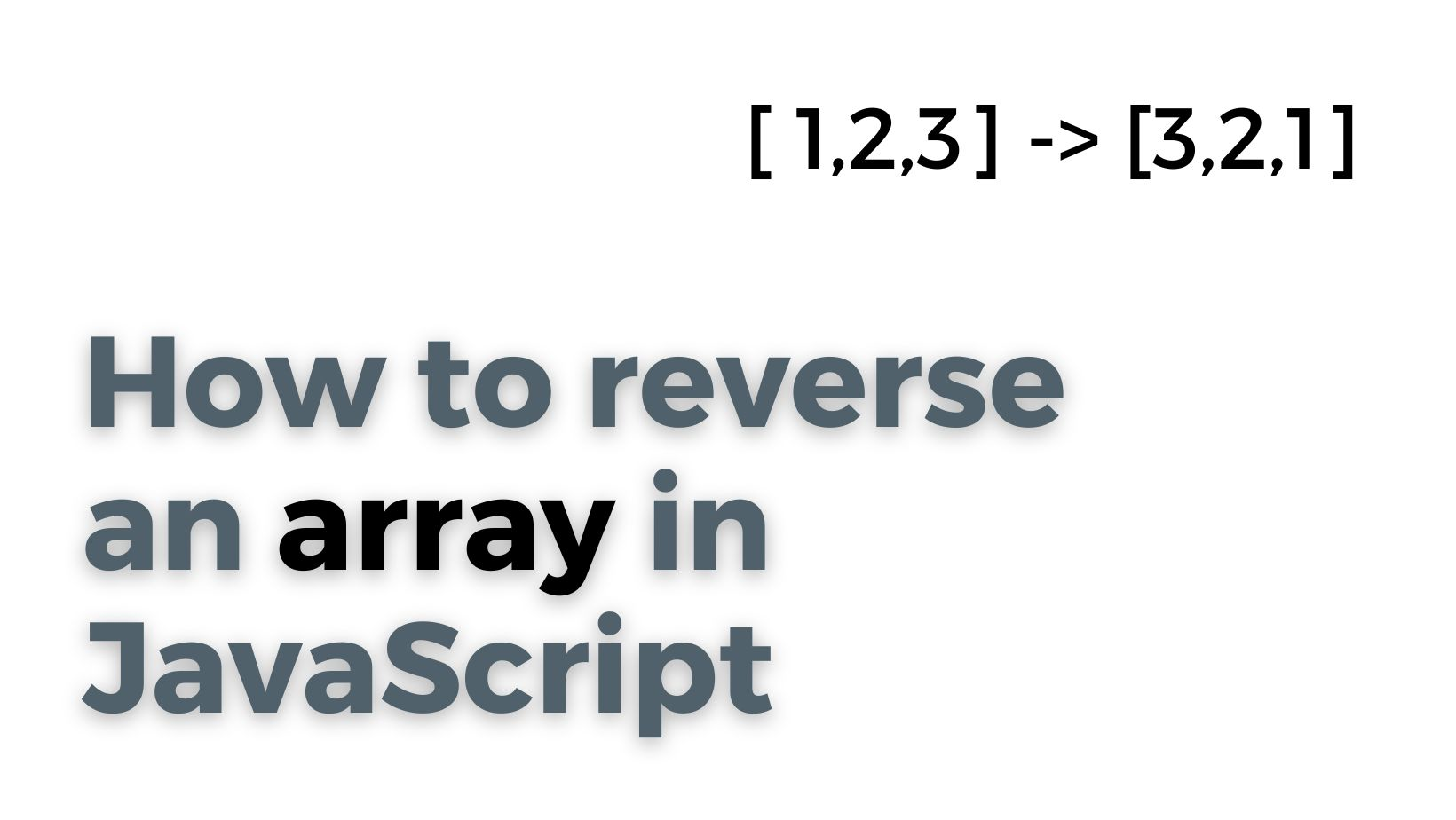# How to reverse an array in JavaScript## Reverse an array by reverse method

To reverse an array in JavaScript, you can use the `reverse()` method. This method modifies the original array, so you'll need to save the result to a new variable if you want to keep the original array intact.

Here's an example of how to use the `reverse()` method to reverse an array:

``````const originalArray = [1, 2, 3, 4, 5];

// Reverse the array
const reversedArray = originalArray.reverse();

console.log(reversedArray);  // Output: [5, 4, 3, 2, 1]``````

## Reverse an array by loop

you can also use a loop to manually reverse the elements in the array. Here's an example of how to do that:

``````const originalArray = [1, 2, 3, 4, 5];

// Create a new empty array
const reversedArray = [];

// Loop through the original array in reverse order
for (let i = originalArray.length - 1; i >= 0; i--) {
// Add each element to the beginning of the new array
reversedArray.unshift(originalArray[i]);
}

console.log(reversedArray);  // Output: [5, 4, 3, 2, 1]``````

Both of these methods will produce the same result, but the `reverse()` method is more concise and easier to read.

## Reverse an array by reduce method

There are a few other ways to reverse an array in JavaScript.

One option is to use the `Array.prototype.reduce()` method to iterate over the elements in the array in reverse order and build a new array with the reversed elements. Here's an example of how to do that:

``````const originalArray = [1, 2, 3, 4, 5];

// Use reduce() to iterate over the array in reverse order
// and build a new array with the reversed elements
const reversedArray = originalArray.reduce((acc, current) => [current].concat(acc), []);

console.log(reversedArray);  // Output: [5, 4, 3, 2, 1]``````

## Reverse an array with JavaScript libraries

There are several libraries that provide methods for reversing arrays in JavaScript.

One such library is the `lodash` library, which provides a `reverse()` method that works similarly to the built-in `Array.prototype.reverse()` method. Here's an example of how to use the `lodash` library to reverse an array:

``````const _ = require('lodash');  // Import the lodash library

const originalArray = [1, 2, 3, 4, 5];

// Reverse the array using the lodash reverse() method
const reversedArray = _.reverse(originalArray);

console.log(reversedArray);  // Output: [5, 4, 3, 2, 1]``````

Another option is the `ramda` library, which provides a `reverse` function that can be used to reverse an array. Here's an example of how to use the `ramda` library to reverse an array:

``````const R = require('ramda');  // Import the ramda library

const originalArray = [1, 2, 3, 4, 5];

// Reverse the array using the ramda reverse() function
const reversedArray = R.reverse(originalArray);

console.log(reversedArray);  // Output: [5, 4, 3, 2, 1]``````

These libraries provide additional functionality beyond just reversing arrays, so they may be a good choice if you need those additional features in your project. However, if you only need to reverse an array, you may prefer to use the built-in `Array.prototype.reverse()` method or one of the other methods mentioned earlier in this conversation.